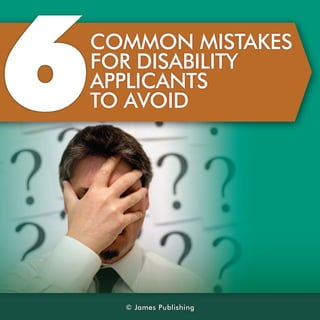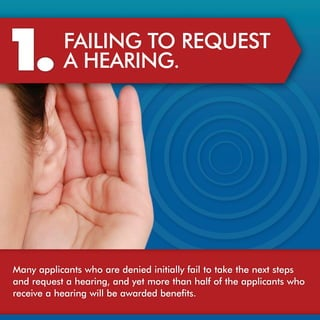Ce diaporama a bien été signalé.
Le téléchargement de votre SlideShare est en cours. ×

# 6 Common mistakes for disability applicants to avoid

Publicité
Publicité
Publicité
Publicité
Publicité
Publicité
Publicité
Publicité
Publicité
Publicité
PublicitéPublicité
Chargement dans…3
×

## Consultez-les par la suite

1 sur 7 Publicité

# 6 Common mistakes for disability applicants to avoid

While you are filling out an application for disability benefits, you should avoid these 6 commonly made mistakes. 1. Failing to request a hearing. 2. Stopping medical treatment. 3. Keeping incomplete records. 4. Filing for unemployment benefits. 5. Using and/ or abusing illegal drugs or alcohol. 6. Failing to get legal representation when needed. These mistakes are made consistently, and being aware of them should give you a leg-up when filing for Social Security disability benefits.

While you are filling out an application for disability benefits, you should avoid these 6 commonly made mistakes. 1. Failing to request a hearing. 2. Stopping medical treatment. 3. Keeping incomplete records. 4. Filing for unemployment benefits. 5. Using and/ or abusing illegal drugs or alcohol. 6. Failing to get legal representation when needed. These mistakes are made consistently, and being aware of them should give you a leg-up when filing for Social Security disability benefits.

Publicité
Publicité

### 6 Common mistakes for disability applicants to avoid

1. 1. 6 C OMMON MI A E S KS T F DS BL Y OR I IT A I A PIA T PL N S C T A OI O V D © J me P bi ig a s u l hn s
2. 2. 1 . F IN T R QU S AL G O E E T I AH A I G E RN . Ma y p la tw oaed ne ii l fit tk ten x s p n a pi ns h r e i nt l alo a e h ett s c d iy a e a drq et h aig a dyt r ta h lo tea pi ns h n e u sa e r , n e moe h n af fh p la tw o n c rci ah aigwlb a ad db n fs ee e e r v n i e w re e ei. l t
3. 3. 2 . SOPI G ME IA T P N DC L TE T N . R A ME T Iy us pt ame t o ,h S c l e ui A miirt nma f o t r t n n w te o i S c ry d ns ai o e a t t o y a s meta y uh v rc v rdo n ln e n e me i l ep su h t o a e e o ee r o o g r e d dc h l. a S e o r o tr e ual a drp ral f o r y tms t a h e y u d c rg lr n e ot lo y u smpo a e c o y v it i rv y u c a c s o s ces ito mpo e o r h n e fr u c s. s
4. 4. 3 . K EI G I C EP N N OMPEE LT RC D . E OR S Ismp r n t d c me t o r dc lo dt n a dal f t i ot to o u n y u me i c n io s n lo ’ a a i y u t ame tA ky u d c r t rc r y u smpo i y u o rr t n. s o r o t s o eod o r y tmsn o r e o t ame teod o e c v i K e y u o nd ti drc r o r t n rc rs n a h it e p o r w eal eod f e s. e al f o rrame t me i t n ,h rp, n a y dc let lo y u t t ns dc i s tea ya d n me i ts e , ao a s rci d eev . e
5. 5. 4 . F IG F IN OR L U E L ME T N MPOY N BN F S E EI . T Wh ny use u e ly n b n fs y uh v t d c r ta e o e k n mpo me t e ei, o a e o e l e h t t a y uaec p beo w rig T id c rt nic nrr t y u o r a a l f okn . hs e l ai s o t y o o r a o a c i t teS c l e ui A miirt nta y uaeu a l t l m o h o i S c ry d ns ai h t o r n be o a a t t o w r.akt y u ds bly a y r eoefigfr n mpo me t ok Tl o o r i it lw e b fr in o u e ly n a i l b c u erc i n u e ly n b n fs hl c i n t b e a s e ev g n mpo me t e ei w i l mig o e i t e a ds be c nb ftloy u ds bly a e i ld a e aa t o r i it c s. a a i
6. 6. 5 . U IG A U IG S /B S N N IE A D U S L G L RG L ORA C OL L OH . Sn eteS c l e ui A miirt nn ln e c n ies rg ic h o i S c ry d ns ai o o g r o s r du a t t o d a dc o o ac h lm c mp n a l ds blis s f r gf m d i in r l oi o e s be i it , uf i r t o s a ie en o o eo teec n io s o lae y u c s. o s o l se n fh s o dt n c mpi ts o r a e Y u h ud e k i c t ame t o a da s i ttl u t y u c s id n . r t n n w n b t n oal ni o r a e s o e e a y l
7. 7. 6 . F IN T G T AL G O E I LG L ER S N A I E A R P E E TT ON WH N N E E . E ED D T edsbly e r giac mpe a dhg -tk s vn. o r h i i h ai s o lx n ihs e ee tY u a i t n a c a cs f en a ad db n fs r s nfa t ice sdwt h n e o b ig w re e ei ae i ic nl n rae i t g i y h po e lg le rsnain Adsbly a yrs f ra l.Mot rp re a rpee tt . i i lw e iaf d be o a i t o s ofr l ns “o t g n fe” fep i o l ite wn f ce t a c ni e te , a e ad ny fh y i. e i n